# Fifth Grade Math Worksheets

### Math instruction for 5th-grade students will focus on developing fluency with addition and subtraction of fractions; developing understanding of multiplication and division of fractions in limited cases; extending division to 2-digit divisors; extending understanding of place-value to decimal fractions; understanding operations with decimals to hundredths; developing fluency with whole-number and decimal operations; and developing an understanding of volume.Students will be thoroughly engaged by these worksheets that review basic mathematical concepts, such as the four operations through six digits. They go on to include word problems, critical thinking, algebra, percentages, ratios, all kinds of graphs with analysis, and measurement. The inspired geometry worksheets will help students become familiar with these topics in fun ways that will easily carry them to higher-level mathematical learning.

Free Fifth Grade Math Worksheets:
Fifth Grade Jumbo Math Worksheets Book - Free PDF
(No Login)
New Math Worksheets for Fifth Graders Each Month

Math Worksheet Practice Workbook
5th Grade Math and Critical Thinking Worksheets

Daily Fifth Grade Math and Critical Thinking Practice

Fifth Grade Daily Homework and Practice Pages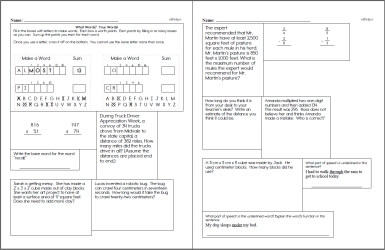Fifth Grade Daily Homework and Practice Pages

Fifth Grade Mixed Review
Level 1: Easiest (First 2 months of school)
Level 2: Next 3 months of school
Level 3: Next 3 months of school
Level 4: Entire Grade (Final months of school)

Weekly Word Problems
Last week's fifth grade math word problems
This week's fifth grade math word problems
Next week's fifth grade math word problems
Daily Review: Customize math review skills with word problems, language arts, and science

edHelperKu Puzzles

Runaway Math Puzzles
 Fifth grade runaway math puzzles Runaway Math Puzzles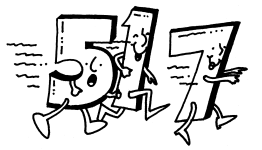Equation Unknowns Puzzle

Place Value

Place Value
Write each number in standard form.
Write each number in two other ways.
Write the place and the value of the underlined digit.
Fill in the missing number.
Write each number in standard form (not in order of place value).
Fill in the missing number (not in order of place value).
Write each number in expanded form using exponents.
Write each number in standard form. (includes exponents)
Write each number in standard form. (decimal place value)
Write each decimal in words. (decimal place value)
Write the value of the underlined digit in words. (decimal place value)
Write the value of the underlined digit in words. (whole numbers and decimal place value)
Place Value Mixed Review

Basic Operations
Addition
Subtraction
Multiplication
Division

Math Facts

Quick Math Facts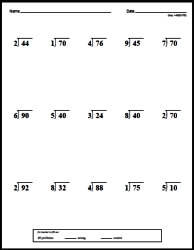Make Quick Math Facts Printable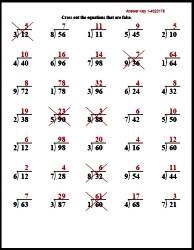Addition
2-3 digits column addition
2-4 digits column addition
4-7 digits column addition
2 digits across addition
2-4 digits across addition
4-5 digits across addition
2 digits with 3 numbers column addition
2-3 digits with 3 numbers column addition
2-5 digits with 3 numbers column addition
Column addition: start with 2 digits and increase by 1 digit in each row
Column addition: start with 2-3 digits and increase by 1 digits every 2 rows
Column addition: start with 2-3 digits and increase by 2 digits every 2 rows
Across addition with 3-5 numbers
Fill in the missing numbers: 2 digits
Fill in the missing numbers: 2-3 digits
Fill in the missing numbers: 4-5 digits
Fill in the missing numbers: 2-6 digits
Math word puzzles
Math addition boxes: 3 x 3
Math addition boxes: 4 x 4
Addition Word Problems (Easier)
Addition Word Problems
Addition Mixed Review

Addition Grouping

Number Bonds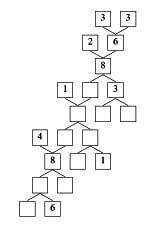Number Bonds

Addition Squares

Super Silly Squares

Subtraction Squares

Locker Combo Math Learning Center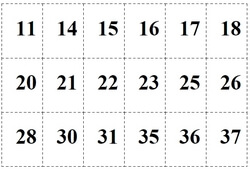Easiest: Open the Locker - Only 1 Number (Grades 4-5) Open the Locker - 2 Numbers in the Locker Combo (Grades 5-6) Open the Locker - 3 Numbers in the Locker Combo (Grades 5-6)

Subtraction
2 digits column subtraction
3 digits column subtraction
2-3 digits column subtraction
4 digits column subtraction
5-6 digits column subtraction
3-5 digits column subtraction
2 digits across subtraction
2-3 digits across subtraction
2-4 digits across subtraction
3-4 digits across subtraction
5-6 digits across subtraction
Column subtraction: start with 2 digits and increase by 1 digit in each row
Column subtraction: start with 2-3 digits and increase by 1 digits every 2 rows
Column subtraction: start with 2-3 digits and increase by 2 digits every 2 rows
Across subtraction with 3-5 numbers
Fill in the missing numbers: 2 digits
Fill in the missing numbers: 2-3 digits
Fill in the missing numbers: 4-5 digits
Fill in the missing numbers: 2-6 digits
Math word puzzles
Math addition boxes: 3 x 3
Math addition boxes: 4 x 4
Subtraction word problems (Easier)
Subtraction word problems
Subtraction Mixed Review

Multiplication
Column Multiplication with Extra Space To Solve Problems
1-2 digits by 1 digit column multiplication
2 digits (and increasing 1 digit every 2 rows) by 1 digit column multiplication
2 digits (and increasing 1 digit every row) by 1 digit column multiplication
Multiples of 10 multiplication (2-5 digits by 1 digit)
2 digits by 2 digits column multiplication
2-3 digits by 2 digits column multiplication
4-5 digits by 2 digits column multiplication
2 digits (and increasing 1 digit every 2 rows) by 2 digit column multiplication
2 digits (and increasing 1 digit every row) by 2 digit column multiplication

Column Multiplication (more problems with less space)
1-2 digits by 1 digit column multiplication
2 digits (and increasing 1 digit every 2 rows) by 1 digit column multiplication
2 digits (and increasing 1 digit every row) by 1 digit column multiplication
Multiples of 10 multiplication (2-5 digits by 1 digit)
2 digits by 2 digits column multiplication
2-3 digits by 2 digits column multiplication
4-5 digits by 2 digits column multiplication
2 digits (and increasing 1 digit every 2 rows) by 2 digit column multiplication
2 digits (and increasing 1 digit every row) by 2 digit column multiplication

Part 2
1-2 digits by 1 digit across multiplication
3-4 digits by 1 digit across multiplication
1-2 digits by 2 digits across multiplication
Across multiplication with 3-5 numbers
Fill in the missing numbers: 1-2 digits
Fill in the missing numbers: 2 digits
Fill in the missing numbers: 2-3 digits
Math multiplication boxes: 3 x 3 (numbers 1-9)
Math multiplication boxes: 4 x 4 (numbers 1-9)
Math multiplication boxes: 3 x 3 (numbers 4-19)
Multiplication word problems (basic)
Multiplication word problems
Multiplication word problems
Multiplication Mixed Review

Multiplying by 3-5 Digits
2-3 digits by 2-3 digits column multiplication
(extra space for work) 2-3 digits by 2-3 digits column multiplication
Multiples of 10 multiplication (2-5 digits by 1 digit)
(extra space for work) Multiples of 10 multiplication (2-5 digits by 1 digit)
3-4 digits by 3-4 digits column multiplication
(extra space for work) 3-4 digits by 3-4 digits column multiplication
2-5 digits by 2-5 digits column multiplication
(extra space for work) 2-5 digits by 2-5 digits column multiplication
Fill in the missing numbers: 3-4 digits
2-3 digits by 3-5 digits across multiplication
Across multiplication with 3-5 numbers (2 Digits)
Multiplication word problems
Multiplication Mixed Review

Division by One-Digit Numbers
Column Division with Extra Space To Solve Problems - Division by One-Digit Numbers
2 digits by 1 digit column division (no remainders)
2-3 digits by 1 digit column division (no remainders)
4-5 digits by 1 digit column division (no remainders)
5-7 digits by 1 digit column division (no remainders)
2 digits by 1 digit column division
2-3 digits by 1 digit column division
4-5 digits by 1 digit column division
5-7 digits by 1 digit column division
2 digits (and increasing 1 digit every 2 rows) by 1 digit column division
2 digits (and increasing 1 digit every row) by 1 digit column division

Column Division (more problems with less space) - Division by One-Digit Numbers
2 digits by 1 digit column division (no remainders)
2-3 digits by 1 digit column division (no remainders)
4-5 digits by 1 digit column division (no remainders)
5-7 digits by 1 digit column division (no remainders)
2 digits by 1 digit column division
2-3 digits by 1 digit column division
4-5 digits by 1 digit column division
5-7 digits by 1 digit column division
2 digits (and increasing 1 digit every 2 rows) by 1 digit column division
2 digits (and increasing 1 digit every row) by 1 digit column division

Part 2 - Division by One-Digit Numbers
Divisibility: complete the table
2 digits by 1 digit across division
2-3 digits by 1 digit across division
4-5 digits by 1 digit across division
2 digits (and increase by 1 digit every row) by 1 digit across division
Fill in the missing numbers: 2 digits by 1 digit
Fill in the missing numbers: 3 digits by 1 digit
Fill in the missing numbers: 4 digits by 1 digit
Division Word Problems
Division Word Problems
Solve equations: 2 digits by 1 digit
Order of operations: 2-3 digits by 1 digit (no parenthesis)
Order of operations: 2-3 digits by 1 digit
Order of operations: 2-4 digits by 1 digit
One-Digit Numbers Division Mixed Review

Division by Two-Digit Numbers
Column Division with Extra Space To Solve Problems - Division by Two-Digit Numbers
Division by multiples of 10: 3-4 digits by 2 digits (no remainders)
Division by multiples of 10: 5-7 digits by 2 digits (no remainders)
Division by multiples of 10: 3-4 digits by 2 digits
3-4 digits by 2 digits column division (no remainders)
4-5 digits by 2 digits column division (no remainders)
5-6 digits by 2 digits column division (no remainders)
6-7 digits by 2 digits column division (no remainders)
3-4 digits by 2 digits column division
4-5 digits by 2 digits column division
5-6 digits by 2 digits column division
6-7 digits by 2 digits column division
3 digits (and increasing 1 digit every 2 rows) by 2 digits column division
3 digits (and increasing 1 digit every row) by 2 digits column division

Column Division (more problems with less space) - Division by Two-Digit Numbers
Division by multiples of 10: 3-4 digits by 2 digits (no remainders)
Division by multiples of 10: 5-7 digits by 2 digits (no remainders)
Division by multiples of 10: 3-4 digits by 2 digits
3-4 digits by 2 digits column division (no remainders)
4-5 digits by 2 digits column division (no remainders)
5-6 digits by 2 digits column division (no remainders)
6-7 digits by 2 digits column division (no remainders)
3-4 digits by 2 digits column division
4-5 digits by 2 digits column division
5-6 digits by 2 digits column division
6-7 digits by 2 digits column division
3 digits (and increasing 1 digit every 2 rows) by 2 digits column division
3 digits (and increasing 1 digit every row) by 2 digits column division

Part 2 - Division by Two-Digit Numbers
Divisibility: complete the table
3-4 digits by 2 digits across division
4-5 digits by 2 digits across division
5-6 digits by 2 digits across division
3 digits (and increase by 1 digit every row) by 2 digits across division
Fill in the missing numbers: 3 digits by 2 digits
Fill in the missing numbers: 4 digits by 2 digits
Fill in the missing numbers: 5 digits by 2 digits
Division Word Problems
Division Word Problems
Solve equations: 3 digits by 2 digits
Solve equations: 4-5 digits by 2 digits
Order of operations: 2-3 digits by 1 digit
Order of operations: 3 digits by 2 digits
Two-Digit Numbers Division Mixed Review

Ordered Pairs and the Coordinate Plane

Graph and Analyze Data
Tally table
Frequency table
Line plots
Stem-and-leaf plots
Vertical bar graphs
Vertical bar graphs (draw graph from table)
Horizontal bar graphs
Horizontal bar graphs (draw graph from table)
Ordered pairs: Write the ordered pair for each point
Ordered pairs: Find each ordered pair. Write the letter for the point named by the ordered pair.
Ordered pairs: Draw the ordered points on the grid. Label each point.
Line graphs
Line graphs (draw graph from table)
Graph and Analyze Data Mixed Review
Stem-and-leaf plots (more difficult)
Vertical bar graphs (more difficult)
Horizontal bar graphs (more difficult)
Graph and Analyze Data Mixed Review

Mean, Median, and Mode

Line Plots
Calculating range from a line plot
Calculating mode from a line plot
Calculating median from a line plot
Calculating mean from a line plot
Mixed

Stem-and-Leaf Plots
Calculating range from a stem-and-leaf plots
Calculating mode from a stem-and-leaf plots
Calculating median from a stem-and-leaf plots
Calculating mean from a stem-and-leaf plots
Mixed

5-7 Numbers
Find the range
Find the mean
Find the median
Find the mode
Find the mean, median, and mode
Find the value
Fine the missing number

8-13 Numbers
Find the range
Find the mean
Find the median
Find the mode
Find the mean, median, and mode
Find the value
Fine the missing number

Review
Mean, Median, and Mode Mixed Review

Algebraic Reasoning
Order of Operations (3-4 numbers, no parenthesis)
Order of operations: simplify
Order of operations: fill in the missing number (1 missing)
Order of operations: fill in the missing operation (1 missing)
Order of operations: fill in all of the numbers
Order of operations: fill in all of the operations
Order of Operations (3-5 digits)
Order of operations: simplify
Order of operations: fill in the missing number (1 missing)
Order of operations: fill in the missing operation (1 missing)
Order of operations: fill in all of the numbers
Order of operations: fill in all of the operations
Algebraic Expressions
Evaluate expressions
More evaluate expressions
Writing algebraic expressions
Solving Equations
Solving addition equations
Solving subtraction equations
Solving multiplication and division equations
Solving addition, subtraction, multiplication, and division equations
Write an equation for each problem. Then solve the equation.
Functions
Complete the function table (rule and easier inputs given)
Complete the function table (rule and inputs given)
Complete the function table (missing some inputs and some outputs)
Write the rule for the function (all the inputs and outputs given)
Write the rule and fill in missing inputs or outputs
Algebraic Expressions Mixed Review
Algebraic Expressions Mixed Review

Number Theory
Divisibility: circle all of the numbers that are divisible by the number
Divisibility: complete the table
Prime or composite
Use the clue to fill in the missing digit
Circle all of the numbers that are multiples of a number
List 3 multiples of each number
Fill in the missing multiple
List all of the factors
Prime factorization
Greatest common factor
Least common multiple
Open-ended number theory questions
Number theory review printable

Understand Fractions
Circle the equivalent fraction
Write two equivalent fractions
Is each fraction in simplest form?
Write each fraction in simplest form
Equivalent fractions: fill in the missing number (easier)
Equivalent fractions: fill in the missing number
Comparing fractions
Ordering fractions (from least to greatest)
Ordering fractions (from greatest to least)
Part of a number
Problem solving
Circle the equivalent improper fraction
Circle the equivalent mixed number
Write each improper fraction as a mixed number
Write each mixed number as an improper fraction
Open-ended fraction problems
Understand Fractions Mixed Review

Add and Subtract Fractions
Add Fractions with Like Denominators
Add fractions with like denominators (horizontal)
Add fractions with like denominators (vertical)
Add fractions with like denominators: find the value of y
Subtract Fractions with Like Denominators
Subtract fractions with like denominators (horizontal)
Subtract fractions with like denominators (vertical)
Subtract fractions with like denominators: find the value of y
Add and Subtract Fractions with Like Denominators
Add and subtract fractions with like denominators (horizontal)
Add and subtract fractions with like denominators (vertical)
Add and subtract fractions with like denominators: find the value of y
Add and Subtract Fractions with Like Denominators (Mixed Numbers)
Add and subtract fractions with like denominators and mixed numbers (horizontal)
Add and subtract fractions with like denominators and mixed numbers (vertical)
Add and subtract fractions with like denominators and mixed numbers: find the value of y
Add Fractions with Unlike Denominators
Add fractions with unlike denominators (horizontal)
Add fractions with unlike denominators (vertical)
Add fractions with unlike denominators: find the value of y
Subtract Fractions with Unlike Denominators
Subtract fractions with unlike denominators (horizontal)
Subtract fractions with unlike denominators (vertical)
Subtract fractions with unlike denominators: find the value of y
Add and Subtract Fractions with Unlike Denominators
Add and subtract fractions with unlike denominators (horizontal)
Add and subtract fractions with unlike denominators (vertical)
Add and subtract fractions with unlike denominators: find the value of y
Add and Subtract Fractions with Unlike Denominators (Mixed Numbers)
Add and subtract fractions with unlike denominators and mixed numbers (horizontal)
Add and subtract fractions with unlike denominators and mixed numbers (vertical)
Add and subtract fractions with unlike denominators and mixed numbers: find the value of y
Problem Solving
Find parts of a number
Addition and subtraction of fractions
Addition and subtraction of fractions (mixed numbers)
Addition and subtraction of fractions (multi-step)
Addition and subtraction of fractions (more problems)
Review
Add and Subtract Fractions Mixed Review

Add and Subtract Decimals
Addition
Column addition of 2 numbers (up to tenths)
Column addition of 2 numbers (up to hundredths)
Column addition of 2 numbers (up to thousandths)
Money column addition (up to \$99.99)
Money column addition (up to \$99,999.99)
Across addition of 2 numbers (up to hundredths)
Across addition of 2 numbers (up to thousandths)

Subtraction
Column subtraction of 2 numbers (up to tenths)
Column subtraction of 2 numbers (up to hundredths)
Column subtraction of 2 numbers (up to thousandths)
Money column subtraction (up to \$99.99)
Money column subtraction (up to \$99,999.99)
Across subtraction of 2 numbers (up to hundredths)
Across subtraction of 2 numbers (up to thousandths)

Mix of Addition and Subtraction
Column addition and subtraction of 2 numbers (up to tenths)
Column addition and subtraction of 2 numbers (up to hundredths)
Column addition and subtraction of 2 numbers (up to thousandths)
Money column addition and subtraction (up to \$99.99)
Money column addition and subtraction (up to \$99,999.99)
Across addition and subtraction of 2 numbers (up to hundredths)
Across addition and subtraction of 2 numbers (up to thousandths)

Part 2
Column addition of 3 numbers
Order of Operations (no parenthesis)
Order of Operations
Order of Operations (one missing number)
Order of Operations (one missing operation)
Order of Operations (fill in all numbers)
Order of Operations (fill in all operations)
Fill in the missing numbers (up to hundredths)
Fill in the missing numbers (up to thousandths)
Math box
Decimal addition and subtraction word problems
Addition and subtraction of decimals: Mixed Review

Multiply and Divide Fractions
Multiply fractions
Multiply fractions: find the value of y
Multiply fractions with mixed numbers
Multiply fractions with mixed numbers: find the value of y
Divide fractions
Divide fractions: find the value of y
Divide fractions with mixed numbers
Divide fractions with mixed numbers: find the value of y
Multiply and divide fractions
Multiply and divide fractions: find the value of y
Multiply and divide fractions with mixed numbers
Multiply and divide fractions with mixed numbers: find the value of y
Multiply and Divide Fractions Mixed Review

Multiply Decimals
Column Multiplication with Extra Space To Solve Problems
Multiply a decimal (up to hundredths) by a Whole Number
Multiply a decimal (up to ten-thousandths) by a Whole Number
Multiply 2 decimals (up to tenths; numbers are less than 1)
Multiply 2 decimals (up to hundredths; numbers are less than 1)
Multiply 2 decimals (up to thousandths; numbers are less than 1)
Multiply 2 decimals (numbers are from 0 to 999.99)

Column Multiplication (more problems with less space)
Multiply a decimal (up to hundredths) by a Whole Number
Multiply a decimal (up to ten-thousandths) by a Whole Number
Multiply 2 decimals (up to tenths; numbers are less than 1)
Multiply 2 decimals (up to hundredths; numbers are less than 1)
Multiply 2 decimals (up to thousandths; numbers are less than 1)
Multiply 2 decimals (numbers are from 0 to 999.99)

Part 2
Across multiplication: a decimal (up to hundredths) by a Whole Number
Fill in the missing numbers (up to tenths)
Fill in the missing numbers (up to hundredths)
Fill in the missing numbers (up to thousandths)
Order of Operations (no parenthesis; numbers up to 9.9)
Order of Operations (no parenthesis; numbers up to 99.99)
Order of Operations
Order of Operations (one missing number)
Order of Operations (one missing operation)
Order of Operations (fill in all numbers)
Order of Operations (fill in all operations)
Decimal multiplication word problems
Multiplication of decimals: Mixed Review

Divide Decimals
Extra Space To Solve Problems
Divide a decimal (up to hundredths) by a whole number with no remainders
Divide a decimal (up to thousandths) by a whole number with no remainders
Divide a decimal (up to hundredths) by a whole number with remainders (give the remainder)
Add Zeros: Divide a decimal (up to hundredths) by a whole number with no remainders
Add Zeros: Divide a decimal (up to hundredths) by a whole number with no remainders
Add Zeros: Divide a decimal (up to hundredths) by a whole number with no remainders
Add Zeros: Larger numbers; dividing by a whole number
Divide a whole number by a decimal
Divide a decimal by a decimal (up to tenths)
Divide a decimal by a decimal (up to hundredths)

More Problems with Less Space
Divide a decimal (up to hundredths) by a whole number with no remainders
Divide a decimal (up to thousandths) by a whole number with no remainders
Divide a decimal (up to hundredths) by a whole number with remainders (give the remainder)
Add Zeros: Divide a decimal (up to hundredths) by a whole number with no remainders
Add Zeros: Divide a decimal (up to hundredths) by a whole number with no remainders
Add Zeros: Divide a decimal (up to hundredths) by a whole number with no remainders
Add Zeros: Larger numbers; dividing by a whole number
Divide a whole number by a decimal
Divide a decimal by a decimal (up to tenths)
Divide a decimal by a decimal (up to hundredths)

Part 2
Across division: divide a decimal (up to thousandths) by a whole number with no remainders
Order of Operations (no parenthesis, up to 9.9)
Order of Operations (no parenthesis, up to 99.9)
Order of Operations
Order of Operations (one missing number)
Order of Operations (one missing operation)
Order of Operations (fill in all numbers)
Order of Operations (fill in all operations)
Decimal division word problems
Division of decimals: Mixed Review

Plane Figures
Name each figure using letters and symbols
Draw and label an example of each
Classify each pair of lines as parallel, intersecting, or perpendicular
Classify each angle as acute, obtuse, straight, or right
Classify polygons
Classify triangles
Classify triangles according to lengths
Circles
Plane Figures Mixed Review

Perimeter

Area

Area and Perimeter
Find the perimeter of each figure
Find the perimeter of each figure using a ruler
Perimeter: Find the value of n
Find the area of each figure
Find the value of n
Find the area and perimeter of each rectangle (no graphics)
Find the perimeter of a complex polygon (composed of 2 rectangles)
Find the perimeter of a complex polygon (composed of 3 rectangles)
Find the perimeter of a complex polygon (composed of 4 rectangles)
Find the area and perimeter of a complex polygon (composed of 2 rectangles)
Find the area and perimeter of a complex polygon (composed of 3 rectangles)
Find the area and perimeter of a complex polygon (composed of 4 rectangles)
Area of a parallelogram (lengths are whole numbers)
Area of a parallelogram (lengths are decimals)
Area of a parallelogram (lengths are fractions)
Area of a triangle (lengths are whole numbers)
Area of a triangle (lengths are decimals)
Area of a triangle (lengths are fractions)
Find the circumference (graphics; lengths are whole numbers)
Find the circumference (graphics; lengths are decimals)
Find the circumference (graphics; lengths are fractions)
Find the circumference (lengths are whole numbers)
Find the circumference (lengths are decimals)
Find the circumference (lengths are fractions)
Area and Perimeter Mixed Review (Grade 5)

Measurement
Customary units of measure
Customary units of measure (with decimals)
Customary units of measure (with fractions)
Metric units of length
Metric units of mass
Metric units of capacity
Metric units of capacity (with metric tons)
Metric units of capacity (mixed - no metric tons)
Metric units of capacity (mixed)
Unit Conversions (chart)
Unit Conversions (convert quantity)
Adding and subtracting customary units of measure
Adding and subtracting customary units of measure (with fractions)
Ordering Metric units
Measurement review printable

Ratios and Proportions
Write each ratio in three ways (graphics)
Write each ratio in three ways
Complete the ratio table (increasing order)
Complete the ratio table (mixed order)
Write five equivalent ratios for each ratio
Write each ratio in simplest form
Proportions: State whether the ratios are proportional (fractions)
Proportions: State whether the ratios are proportional (mixed ways of writing ratios)
Proportions: Find the missing number (fractions)
Proportions: Find the missing number (mixed ways of writing ratios)
Create a proportion (4 numbers given)
Create a proportion (5 numbers given)
Create a proportion (6 numbers given)
Fill in the blank to complete the proportion
Find the unit rate
Fill in the blank to complete the proportion (more difficult)
Better buys
Similar figures
Ratios and Proportions Review Printable

Percents
Write the percent of the shaded portion in each grid
Write each fraction as a percent
Write each decimal as a percent
Write each ratio as a percent
Write each fraction, decimal, or ratio as a percent
Write the percent as a fraction
Write the percent as a decimal
Write the percent as a ratio
Algebra: Fill in the missing number
Order 3 numbers from least to greatest
Order 3 numbers from greatest to least
Order 6 numbers from least to greatest
Order 6 numbers from greatest to least
Find 10% of each number
Find the percent of each number (multiples of 5%)
Find the percent of each number
Percent of numbers: Find the missing number
Open-ended questions
Circle graphs
Percents Mixed Review

Beginning Algebra
Evaluate expressions
Writing algebraic expressions
Writing algebraic expressions (compound expressions)
Combining like terms
Solving addition equations
Solving subtraction equations
Solving addition and subtraction equations (including 3+ terms)
Solving multiplication and division equations
Solving equations (mix)
Beginning Algebra Review Printable

Coordinate Graphing
Coordinate Plane and Functions
Write the coordinates for each point
Finding ordered pairs
Drawing ordered pairs
Naming quadrants
Complete the function table
Complete the function table and then graph the function
Make a function from a line
Mixed Review
Transformations
Translations
Reflections
Rotations
Mix of translations, reflections, and rotations
Do multiple translations at once
Review
Coordinate Graphing Mixed Review

Test Prep - Concepts
from the test prep section, requires registration
Numeration
Number Concepts
Properties
Fractions and Decimals
Review of Number Concepts

Test Prep - Computation
from the test prep section, requires registration
Addition
Subtraction
Multiplication
Division
Review of Computation

Test Prep - Applications
from the test prep section, requires registration
Geometry
Measurement
Problem Solving - Word Problems!
Problem Solving Applications (Charts, etc)

Have a suggestion or would like to leave feedback?
Leave your suggestions or comments about edHelper!